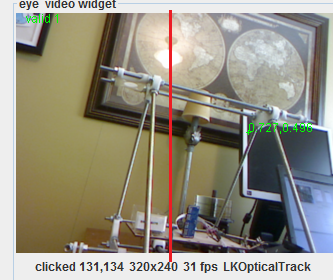# Servo Stickyness in TrackingThis following code is used to limit the Y servo in the Tracking service.  Previously the first line did not exist an the PID value would be computed even if the servo limit did not allow it to move.  This would make a "very" sticky problem because the computed PID value would grow and grow and if the target was in a valid range - it would take the same amount of time (or greater) to get unstuck as the time it was stuck.

Now, however, the PID value is not computed when the servo has hit a limit (a good thing) -
The servo is not given the opportunity to move (bad thing)

It should move but only back to a valid position - it should not move or compute PID value for a position which would put it "more" into the invalid range

So logic will be needed to see if the new possible position is in the valid region - then it should be allowed to compute PID and move - otherwise write the error.

```if ((currentYServoPos <= ymin && ySetpoint - targetPoint.y < 0) || (currentYServoPos >= ymax && ySetpoint - targetPoint.y > 0)) {
error(String.format("%d y limit out of range", currentYServoPos));
} else {
if (ypid.compute()) {
computeY = ypid.getOutput();
currentYServoPos += (int) computeY;
if (currentYServoPos != lastYServoPos) {
y.moveTo(currentYServoPos);
currentYServoPos = y.getPosition();
lastYServoPos = currentYServoPos;
}
} else {
log.warn("y data under-run");
}
}```

## Comment viewing options